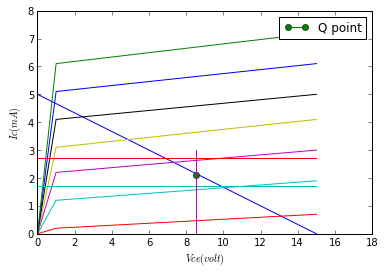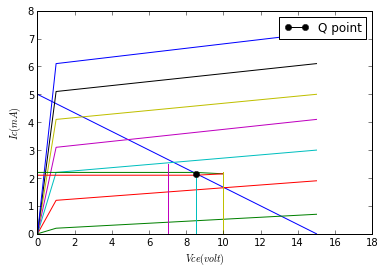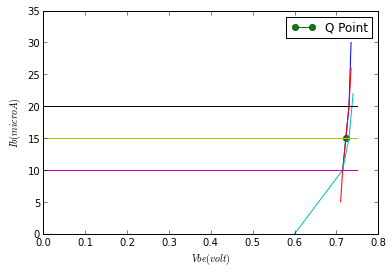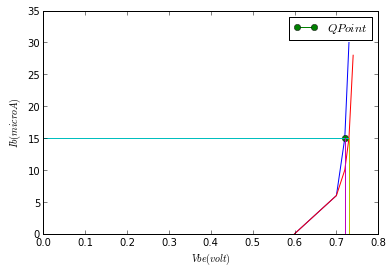# Chapter 8:Small Signal Amplifiers¶

### Example 8.1 Page no.271¶

In :
#Given
Ic=2*10**(-3)                                         #A, collector current
Vce=8.5                                                   #V, collectoe emitter voltage

#Calculation
#hfe=delta(ic)/delta(ib),                      #forward current ratio in CE mode
#Vce=constant                                    #collector emitter voltage
hfe=(2.7-1.7)*10**(-3)/((20-10)*10**(-6))
#hoe=delta(ic)/delta(Vce),                #output admittance in CE mode
#ib=constant
hoe=(2.2-2.1)*10**(-3)/(10-7)
#hie=delta(Vbe)/delta(ib),               #dynamic input resistance
#Vce=constant
hie=(0.73-0.715)/((20-10)*10**(-6))
#hre=delta(Vbe)/delta(Vce),ib=constant       #reverse voltage ratio in CE mode
hre=(0.73-0.72)/(20-0)

#Result
print "hfe =  ",hfe
print "hoe =  ",round(hoe/10**(-6),2),"microS"
print "hie =  ",hie/10**3,"kohm"
print "hre =  ",hre

#a) Plot
Vce0=[0,15]
Ic0=[5,0]
a0=plot(Vce0,Ic0)
q1=plot(8.5,2.1,label='Q point',marker='o')
legend()
# AT Ib=0 microA
Vce1=[0,1,15]
Ic1=[0,0.2,0.7]
a1=plot(Vce1,Ic1)
# AT Ib=10 microA
Vce2=[0,1,15]
Ic2=[0,1.2,1.9]
a2=plot(Vce2,Ic2)
# AT Ib=20 microA
Vce3=[0,1,15]
Ic3=[0,2.2,3]
a3=plot(Vce3,Ic3)
# AT Ib=30 microA
Vce4=[0,1,15]
Ic4=[0,3.1,4.1]
a4=plot(Vce4,Ic4)
# AT Ib=40 microA
Vce5=[0,1,15]
Ic5=[0,4.1,5]
a5=plot(Vce5,Ic5)
# AT Ib=50 microA
Vce6=[0,1,15]
Ic6=[0,5.1,6.1]
a6=plot(Vce6,Ic6)
# AT Ib=60 microA
Vce7=[0,1,15]
Ic7=[0,6.1,7.2]
a7=plot(Vce7,Ic7)
#At Vce=8.5 V
Vce8=[0,15]
Ice8=[2.7,2.7]
a8=plot(Vce8,Ice8)

Vce9=[0,15]
Ice9=[1.7,1.7]
a9=plot(Vce9,Ice9)
#at Vce=8.5
Vce10=[8.5,8.5]
Ice10=[0,3]
a10=plot(Vce10,Ice10)
xlim(0,18)
ylim(0,8)
xlabel("$Vce(volt)$")
ylabel("$Ic(mA)$")
show(q1)
show(a0)
show(a1)
show(a2)
show(a3)
show(a4)
show(a5)
show(a6)
show(a7)
show(a8)
show(a9)
show(a10)

#(b) Plot
#Plot
Vce0=[0,15]
Ic0=[5,0]
a0=plot(Vce0,Ic0)
# AT Ib=0 microA
Vce1=[0,1,15]
Ic1=[0,0.2,0.7]
a1=plot(Vce1,Ic1)
# AT Ib=10 microA
Vce2=[0,1,15]
Ic2=[0,1.2,1.9]
a2=plot(Vce2,Ic2)
# AT Ib=20 microA
Vce3=[0,1,15]
Ic3=[0,2.2,3]
a3=plot(Vce3,Ic3)
# AT Ib=30 microA
Vce4=[0,1,15]
Ic4=[0,3.1,4.1]
a4=plot(Vce4,Ic4)
# AT Ib=40 microA
Vce5=[0,1,15]
Ic5=[0,4.1,5]
a5=plot(Vce5,Ic5)
# AT Ib=50 microA
Vce6=[0,1,15]
Ic6=[0,5.1,6.1]
a6=plot(Vce6,Ic6)
# AT Ib=60 microA
Vce7=[0,1,15]
Ic7=[0,6.1,7.2]
a7=plot(Vce7,Ic7)

#At Vce=8.5 V
Vce8=[0,8.5,10]
Ice8=[2.2,2.2,2.15]
a8=plot(Vce8,Ice8)
# and
Vce9=[0,8.5,10]
Ice9=[2.1,2.1,2.15]
a9=plot(Vce9,Ice9)
#at Vce=8.5
Vce10=[8.5,8.5]
Ice10=[0,2.2]
a10=plot(Vce10,Ice10)

#at Vce=7 V
Vce11=[7,7]
Ice11=[0,2.5]
a11=plot(Vce11,Ice11)
#at Vce=10 V
Vce12=[10,10]
Ice12=[0,2.2]
a12=plot(Vce12,Ice12)

q2=plot(8.5,2.15,marker='o',label='Q point')
legend()

xlim(0,18)
ylim(0,8)
xlabel("$Vce(volt)$")
ylabel("$Ic(mA)$")
show(a0)
show(q2)
show(a1)
show(a2)
show(a3)
show(a4)
show(a5)
show(a6)
show(a7)
show(a8)
show(a9)
show(a10)
show(a11)
show(a12)

#C) plot
#at Vce=0 V
Vbe1=[0.715,0.730,0.735]
Ib1=[10,20,30]
ab1=plot(Vbe1,Ib1)
q3=plot(0.722,15,marker='o',label='Q Point')
legend()

#at Vce=8.5 V
Vbe2=[0.710,0.715,0.730,0.735]
Ib2=[5,10,20,26]
ab2=plot(Vbe2,Ib2)

#at Vce=20 V
Vbe3=[0.6,0.715,0.730,0.740]
Ib3=[0,10,15,22]
ab3=plot(Vbe3,Ib3)

#at Ib=10 microA
Vbe4=[0,0.7,0.75]
Ib4=[10,10,10]
ab4=plot(Vbe4,Ib4)

#at Ib=15 microA
Vbe5=[0,0.7,0.75]
Ib5=[15,15,15]
ab5=plot(Vbe5,Ib5)
#at Ib=20microA
Vbe6=[0,0.7,0.75]
Ib6=[20,20,20]
ab6=plot(Vbe6,Ib6)

xlim(0,0.8)
ylim(0,35)
xlabel("$Vbe(volt)$")
ylabel("$Ib(microA)$")
show(ab1)
show(q3)
show(ab2)
show(ab3)
show(ab4)
show(ab5)
show(ab6)

#d) plot
#at Vce=0 V
Vbe1=[0.6,0.7,0.72,0.73]
Ib1=[0,6,15,30]
ab_1=plot(Vbe1,Ib1)
q4=plot(0.72,15,marker='o',label='$Q Point$')
legend()
#Vce=20 V
Vbe2=[0.6,0.7,0.72,0.73,0.74]
Ib2=[0,6,10,15,28]
ab_2=plot(Vbe2,Ib2)
#At Ib=15 microA
Vbe3=[0,0.72,0.73]
Ib3=[15,15,15]
ab_3=plot(Vbe3,Ib3)

#At Vbe=0.72
Vbe4=[0.72,0.72]
Ib4=[0,15]
ab_4=plot(Vbe4,Ib4)
#At Vbe=0.73
Vbe5=[0.73,0.73]
Ib5=[0,15]
ab_5=plot(Vbe5,Ib5)

xlim(0,0.8)
ylim(0,35)
xlabel("$Vbe(volt)$")
ylabel("$Ib(microA)$")
show(ab_1)
show(q4)
show(ab_2)
show(ab_3)

hfe =   100.0
hoe =   33.33 microS
hie =   1.5 kohm
hre =   0.0005### Example 8.2 Page no.276¶

In :
ri=2000.0                                                            #Ohms, input resistance
Rb=150000.0                                                     #Ohms, base resistance

#Calculation
Zin=Rb*ri/(Rb+ri)

#result
print " The Input Impedance of the Amplifier is Zin =  ",round(Zin/10**3,3),"kohm"

#(b)find the  voltage gain (Av)
Beeta=100
ri=2000.0                                                        #Ohms
Rac=5000.0                                                    #Ohms Resistance on outputside

#Calculation

Av=Beeta*Rac/ri
#result

print " The Voltage Gain of the Amplifier with phase of pi/2  is ",Av
#(c) find the current gain  (Ai)
#Let input Current ib=2A
ib=2                                                                     #A, Assumption
io=100*ib

#Calculation
Ai=io/ib                                                              # Current Gain
#result
print "The Current Gain of the Amplifier is Ai = ",Ai

 The Input Impedance of the Amplifier is Zin =   1.974 kohm
The Voltage Gain of the Amplifier with phase of pi/2  is  250.0
The Current Gain of the Amplifier is Ai =  100


### Example 8.3 Page no.277¶

In :
Bac=150.0                                     #ac current gain
rin=2000.0                                    #Ohms, input resistance
R1=4700.00                                    #Ohms, resistance
R2=12000.0                                    #Ohms  resistance

#Calculation
Rac=R1*R2/(R1+R2)                             # ohm , resistance
Av=Bac*Rac/rin
#result
print "a"
print "the Voltage Gain of the Amplifier with phase of pi/2 is",round(Av,1)

#(b)find input impedance
R3=75000.0                                 #Ohms
R4=7500.00                                 #Ohms

#Calculation
Zin=R3*R4*rin/(R3*R4+R4*rin+rin*R3)

#result
print "b"
print "The Input Impedance of the Amplifier is Zin =  ",round(Zin/10**3,1),"kohm"

#(c)Q point

Vcc=15                                     #V
R1=75000.0                                 #Ohms
R2=7500.00                                 #Ohms
Rc=4700.0                                  #Ohms
Re=1200.0                                  #Ohms

#Calculation
Vb=Vcc*R2/(R1+R2)                          #V, base voltage
Ve=Vb
Ie=Ve/Re                                   #A, emitter current
Vce=Vcc-(Rc+Re)*Ie                         #V, collector emitter voltage
#result
print "c"
print "voltage at the base is ",round(Vb,2),"V"
print "Emitter current is ",round(Ie/10**(-3),2),"mA"
print "The collector to emitter voltage  is",round(Vce,2),"v"

a
the Voltage Gain of the Amplifier with phase of pi/2 is 253.3
b
The Input Impedance of the Amplifier is Zin =   1.5 kohm
c
voltage at the base is  1.36 V
Emitter current is  1.14 mA
The collector to emitter voltage  is 8.3 v


### Example 8.4 Page no.283¶

In :
u=20                              #amplification factor
rp=10*10**3                       #Ohms, resistance
#Calculation
A=u*Rl/(rp+Rl)                   #V, voltage gain
#result
print " The Voltage Gain of the Amplifier  with phase of pi/2 is",A

 The Voltage Gain of the Amplifier  with phase of pi/2 is 10


### Example 8.5 Page no.286¶

In :
gm=3000*10**(-6)                     #S, transconductance
Rl=22*10**3                              #Ohms, resistance
rp=300*10**3                            #Ohms, resistance

#Calculation
#A=-(gm*Rl/(1+(Rl/rp))), For rp>>Rl we get
A=gm*Rl                                   #with Phase of 180 degrees
# Results
print "The Gain of the Amplifier   with phase of pi/2 is",A

The Gain of the Amplifier   with phase of pi/2 is 66.0


### Example 8.6 Page no.286¶

In :
Rl=12000.0                          #Ohms, load resistance
Rg=1000000.0                    #Ohms, given resistance
Rs=1*10**3                      #Ohms, given resistance
Cs=25*10**(-6)                #F. capacitance
u=20                                    #amplification factor
rd=10**5                      #Ohms, dynamic drain resistance
vi=0.1                                 #V, input voltage
f=1*10**3                         #Hz, frequency

#Calculation
import math
Xcs=1/(2*math.pi*f*Cs)
#As Xcs comes out to be much smaller than Rs, Rs is completely bypassed
A=u*Rl/(Rl+rd)
vo=A*vi
# Result
print " The Output Signal Voltage of the Amplifier is vo = ",round(vo,3),"v"

 The Output Signal Voltage of the Amplifier is vo =  0.214 v